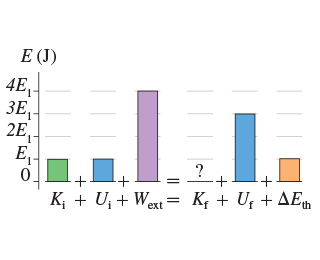# Problem: Consider the process shown in the figure below. Suppose E1 = 3 J. What is the final kinetic energy of the system for the process shown in the figure?

🤓 Based on our data, we think this question is relevant for Professor Demchenko's class at VCU.

###### Problem Details

Consider the process shown in the figure below. Suppose E1 = 3 J. What is the final kinetic energy of the system for the process shown in the figure?